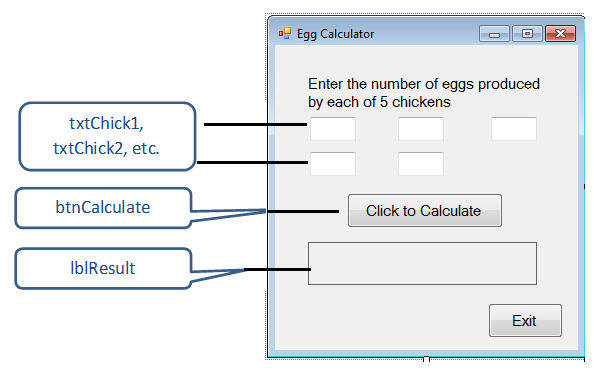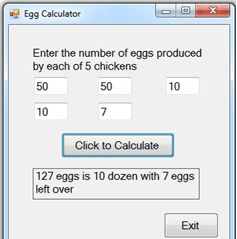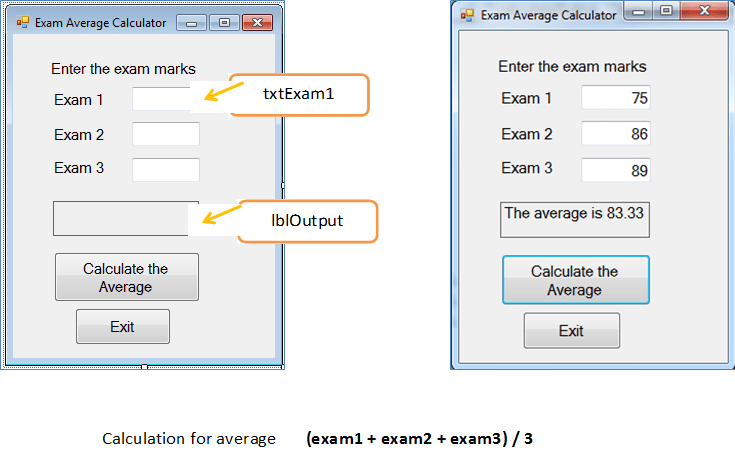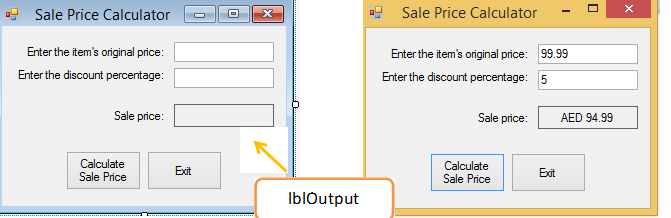# Exercise-1: Processing Data

### Practice Exercices

#### Eggs GUI

Write a program (EggsGUI) that allows the user to input the number of eggs produced in a month by each of 5 chickens. Calculate the total number of eggs, then display the total in dozens and eggs left over. For example, a total of 127 eggs is 10 dozen and 7 eggs left over. See interface here:Results when program is run using test data:To calculate and display the total, dozens, and eggs left over the following steps are needed:

Declare the variables needed
int eggs1, eggs2, eggs3, eggs4, eggs5;
int total, dozens, eggsLeftOver;

Get user input:
eggs1 = int.Parse(txtChick1.Text); repeat this for each of the text boxes

Calculate the total, dozens, and eggs left over
total = eggs1 + eggs2 + eggs3 + eggs4 + eggs5;
dozens = total/12;
eggsLeftOver = total%12;

Display the results lblResult.Text = total.ToString() + " eggs is " + dozens.ToString() + " dozen with " + eggsLeftOver.ToString() + " eggs left over";

#### GUI program (Exams)

Write a GUI program (Exams) that allows the user to input the scores for 3 exams. Display the average of the exam scores to two decimal places.
Here is the interface:#### GUI program (SalePrice)

Write a GUI program (SalePrice) that allows the user to input the item’s original price and discount percentage.
Display the sale price in the output label to two decimal places as it is shown here: